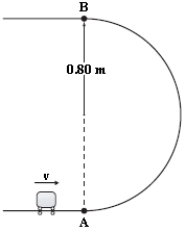# [Solved] A 1

Question 15
Multiple Choice

## A 1.2-kg mass is projected up a rough circular track (radius = 0.80 m) as shown. The speed of the mass at point A is 8.4 m/s, and at point B, it is 5.6 m/s. What is the change in mechanical energy between A and B caused by the force of friction?A)−2.7 J
B)−8.8 J
C)−4.7 J
D)−6.7 J
E)−19 J

10+ million students use Quizplus to study and prepare for their homework, quizzes and exams through 20m+ questions in 300k quizzes.

### Physics & Astronomy

Explore our library and get Introduction to Physical Science Homework Help with various study sets and a huge amount of quizzes and questions

120

Study sets

3.1K

Quizzes

233.2K

Questions

Upload material to get free accessInvite a friend and get free accessSubscribe and get an instant access